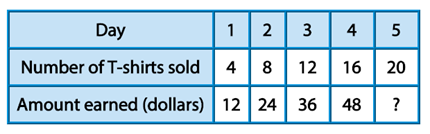Mathematics
Easy

Question

# The table shows two sequences of numbers.The rule that describes the number of T-shirts sold relates to the amount earned is....## multiply by 5add 10multiply by 3divide by 5Hint:

## The correct answer is: multiply by 3

### So as we know that a pattern or sequence in a series of numbers is called a number pattern. Generally, this pattern builds a connection between all integers.Some numerological pattern types are: There are two typical patterns for number sequences: Arithmetic Sequences Geometric Sequences The following are some examples of Number Patterns' unique sequences: Square Numbers Cubic Sequence Triangular Numbers Fibonacci Numbers We have been given 4, 8, 12, 16, 20 and 12, 24, 36, 48, 60. Now looking at this pattern we can identify that the number is added from each and hence the order is ascending.This pattern follows x2 from the previous term in both the series. The rule that describes the number of T-shirts sold relates to the amount earned is multiplied by 3.4 x 3 = 128 x 3 = 2412 x 3 = 3616 x 3 = 4820 x 3 = 60

Here we were given two number series and we were supposed to find the rule that describes the number of T-shirts sold relates to the amount earned. There are numerous different sorts of number patterns. They could be a series of even or odd numbers, multiples of a specific number, ascending or descending, etc. The rule that describes the number of T-shirts sold relates to the amount earned is multiplied by 3.

### Related Questions to study#### With Turito Foundation.#### Get an Expert Advice From Turito.